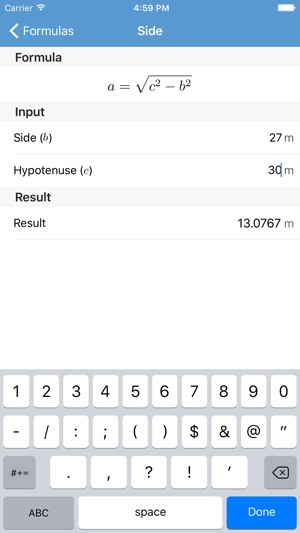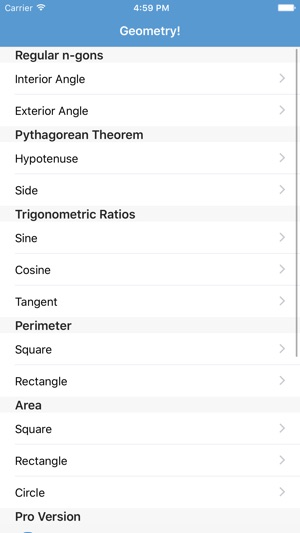Screenshots

••Description

Geometry! and Geometry! Pro are based on the the same engine which powers Physics! and Physics! Pro.

Geometry! is a geometry calculator which can calculate:

+ Measure of each interior or exterior angle of a regular n-gon

+ The Pythagorean Theorem

+ sin, cos, and tan of A

+ Perimeter of a
+ Square
+ Rectangle

+ Area of a
+ Square
+ Rectangle
+ Circle

The full version of this app is Geometry! Pro. In Geometry! Pro you get more formulas:

+ Perimeter of a
+ Triangle
+ Circle

+ Area of a
+ Parallelogram
+ Triangle
+ Trapezoid
+ Equilateral Triangle
+ Regular Polygon

+ Surface Area of a
+ Right Prism
+ Right Cylinder
+ Regular Pyramid
+ Right Cone
+ Sphere

+ Volume of a
+ Cube
+ Cylinder
+ Pyramid
+ Prism
+ Cone
+ Sphere

Also, in Geometry! Pro, just like Physics! Pro, you can add your own custom formulas!

Also, try the Physics! app for any physics formula calculation.

What’s New

Version 3.0

Rebuilt the app from the ground up.

Information

Seller
Paul Erway
Size
13.8 MB
Category
Education
Compatibility

Requires iOS 8.0 or later. Compatible with iPhone, iPad and iPod touch.

Languages

English

Age Rating
Rated 4+
Price
Free

Supports

•